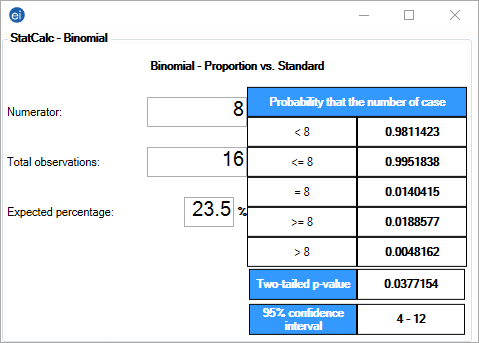# StatCalc: Statistical Calculators

### Binomial

The binomial distribution states the probability that a number of positive outcomes occurs given the expected percentage of positive outcomes and the total number of observations taken.

#### Example

Suppose 23.5% of individuals in a given patient population are HIV positive. If 16 individuals are selected from this patient population, what are the chances that at least eight of these individuals are HIV positive?

1. From the Epi Info™ main page, select StatCalc.
2. Select Population Binomial (Proportion vs Std). The Unmatched Case-Control window opens.
3. Enter the Numerator as 8.  This is the number of patients with the disease.
4. Enter the Total Observations as 16.  This is the patient population of the study.
5. Enter the Expected Percentage as 23.5.  This is the expected percentage of cases infected with a particular disease.Figure 10.15: Binomial Distribution

Based on the inputs for this binomial distribution, there is a 1.9% chance that eight or more of the 16 patients are HIV positive. Additional percentages are stated for the ranges outlined in the left column of the table.

Page last reviewed: September 16, 2022, 12:00 pm